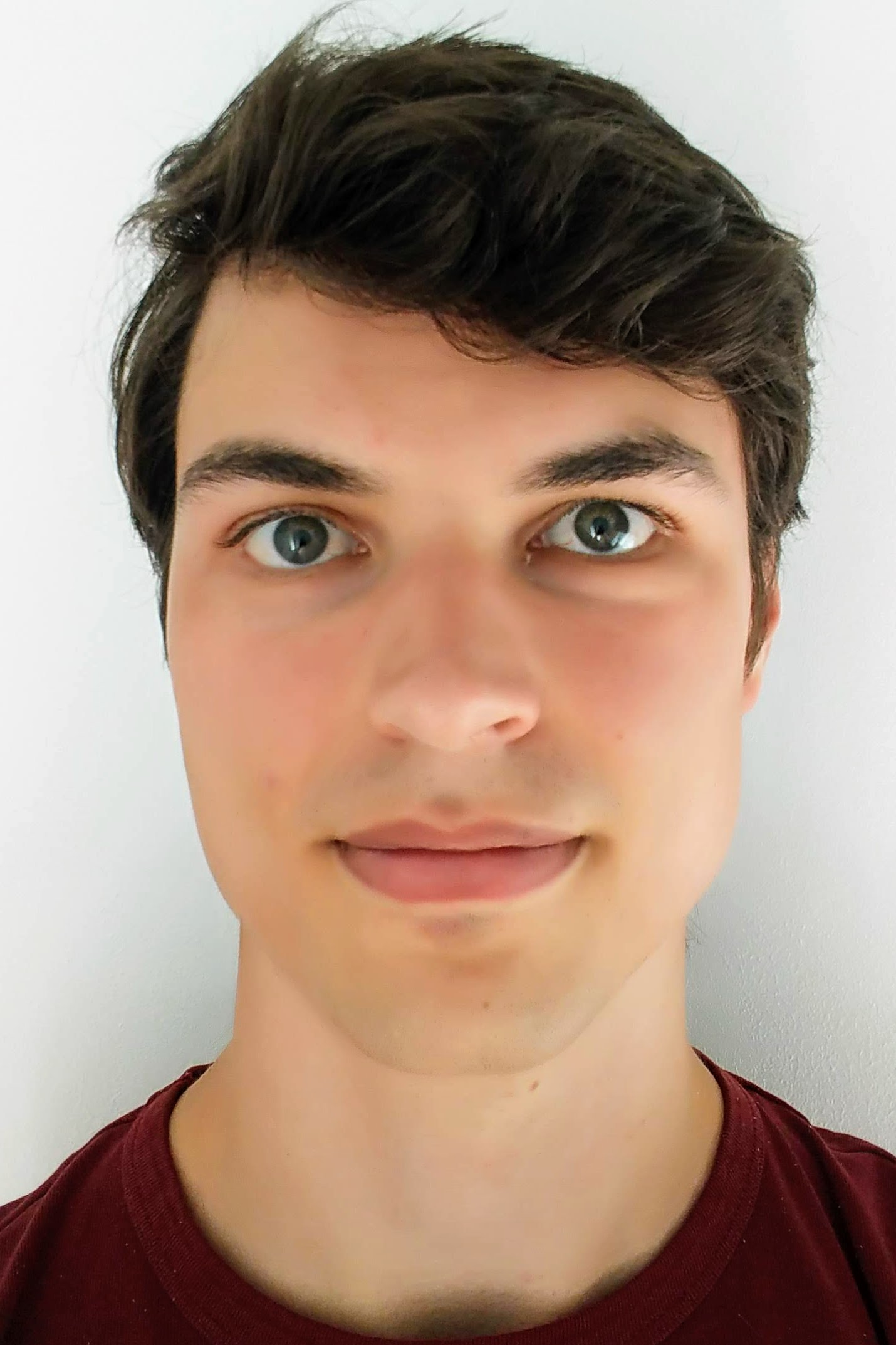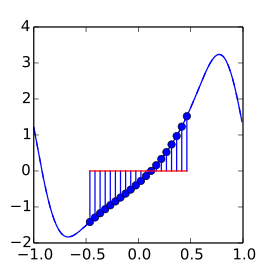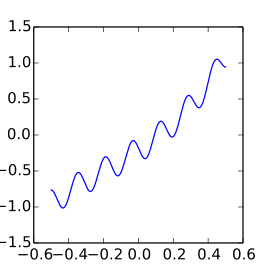I am a researcher at the KU Leuven Computer Science department after obtaining a PhD at KU Leuven under supervision of Daan Huybrechs. My research is on algorithms for frame approximations and their applications.

Numerical Analysis and Applied Mathematics Section
Celestijnenlaan 200A - box 2402
B-3001 Heverlee (Leuven)
Belgium

Room : 02.49
roel.matthysen@kuleuven.be

CV

last update 05/2018

## Research

My PhD research is on function approximation using frames with certain eigenvalue distributions. These arise when, for example, restricting a Fourier Basis on a rectangular domain to some smaller enclosed domain. This results in an accurate approximation of the function on the smaller domain, that is defined on the larger (and easier to work with) domain.### Function Values

We start from function values that are given on an equispaced grid defined on an arbitrary domain.

Example: data samples from the function $$f(x)=10e^x-17-10\cos(x-2.3)$$### Extension

Our algorithm finds a function approximation that is periodic on some bounding box.

Example: An extension of $$f(x)$$ from $$[-0.5,0.5]$$ to $$[-1,1]$$.### Applications

The resulting extension is used to easily solve problems such as this Helmholtz differential equation.

Example: the 1D Helmholtz equation $$\Delta p(x) + 40^2 p(x) = 1000f(x)$$ with homogeneous Neumann boundary conditions.

Helmholtz equation simulated in a star-shaped domain with a hole, for a point source moving from left to bottom. Our algorithms computes an approximate decomposition to the embedded Fourier problem matrix, that can be calculated and applied more efficiently than full singular value decompositions.
Wave equation simulated on a star-shaped domain, with Gaussian initial value. The efficiency of applying the decomposition comes to play when solving the boundary-value problem in each iteration.

## Publications, talks and posters

• #### PhD Defense

March 19, 2018, 17h00

Aula van de Tweede Hoofdwet, Thermotechnisch Instituut
thesis slides
• #### SIAM Journal on Numerical Analysis paper

May, 2017

Function approximation on arbitrary domains using Fourier extension frames
Technical report
• #### FoCM 2017

July 11, 2017

Poster at the 2017 Foundations of Computational Mathematics conference in Barcelona.
pdf
• #### AT15 Proceedings paper

December, 2016

Computing with functions on domains with arbitrary shapes
Technical report
• #### Approximation Theory 15

May 23, 2016

Presentation at the Approximation Theory 15 conference in San Antonio, Texas.
slides
• #### NATW Seminar series talk

April 14, 2016

Presentation in the NATW Seminar series at the KU Leuven Computer Science department.
slides
• #### SIAM Journal of Scientific Computing Paper

March 17, 2016

Fast algorithms for the computation of Fourier Extensions of arbitrary length
SIAM J. Sci. Comput.
Vol. 38, No.2, pp. A899-A922
• #### New Directions in Numerical Computation

August 25, 2015

Contributed talk at the New Directions in Numerical Computations conference, held in celebration of Nick Trefethen's 60th birthday at the Mathematical Institute of Oxford University.
slides
• #### SIAM CSE 2015

March 16, 2015

Poster at the 2015 SIAM conference on Computational Science and Engineering in Salt Lake City.
pdf
• #### Research cluster at ICERM

November 6, 2014

Research talk as invited participant at the research cluster Computational Challenges in Sparse and Redundant Representations. Held at the Institute for Computational and Experimental Research in Mathematics (ICERM) at Brown University.
slides
• #### Woudschoten 2014

October 9, 2014

Poster at the 2014 Woudschoten conference of the Werkgemeenschap Scientific Computing held in Zeist, The Netherlands.
pdf
• #### ICOSAHOM 2014

June 23, 2014

Contributed talk at the 2014 International Conference on Spectral And High Order Methods.
slides
• #### MCQMC 2014

April 7, 2014

Contributed talk at the 2014 International Conference on Monte Carlo and Quasi Monte Carlo methods, on research done during my Master's thesis.
slides